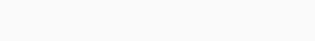# Wine Quality Prediction using Machine Learning in Python

Prediction for the quality of any product is an interesting matter to know about the product in detail and everyone interested to know more about the product quality and their contents. For this here we take one example of wine quality by using Machine Learning in Python.

## Building predictor for wine quality prediction

We build the prediction of wine quality and here their predictor made in four steps

#### Step-1 Importing required libraries

Here we are using libraries like Pandas for reading data and performing an operation on data, Sklearn for modeling operations, Seaborn for visualizing the data.

```import pandas as pd
import numpy as np
import seaborn as sns
from sklearn.preprocessing import StandardScaler
from sklearn.model_selection import train_test_split
from sklearn.tree import DecisionTreeClassifier
from sklearn.ensemble import RandomForestClassifier
from sklearn.metrics import accuracy_score```

#### Step-2 Reading the data from csv files

```wine_data=pd.read_csv("winequality-red.csv")

Output:-Count plot of the wine data of all different qualities.

`sns.countplot(x='quality',data=wine_data)`

Output:To get more information about data we can analyze the data by visualization for example plot for finding citric acid in different types of quality of the wine.

`sns.barplot(x='quality',y='citric acid',data=wine_data)`

Output:Step-3 Splitting and scaling the data

Now we get X as input and y as a target of data than splitting data into train and test data.

```X=wine_data.drop("quality",axis=1)
y=wine_data['quality']
X_train,X_test,y_train,y_test=train_test_split(X,y,test_size=0.30,random_state=51)```

Scaling and transforming data.

```SC=StandardScaler()
X_train = SC.fit_transform(X_train)
X_test = SC.fit_transform(X_test)```

#### Step-4 making model and predict from it

Initializing the model and fitting training data into it. Here, we use a Random forest classifier.

```RFC= RandomForestClassifier(n_estimators=200)
RFC.fit(X_train, y_train)```

Predicting data for test data.

`y_pred = RFC.predict(X_test)`

Finding the accuracy of the model.

```accuracy=accuracy_score(y_test,y_pred)
print('accuracy of the model is {:.2f}% '.format(accuracy*100))```

Output:-Dataset

The data set used here is for the wine quality dataset. It is available on Kaggle. You can download it from here: Wine Quality Dataset

#### Conclusion

From this model of the prediction for wine quality not only we get the quality of the wine with approx 68% of the accuracy. This type of model use to find the quality of the other any product with set it’s relevant dataset and find the quality of that product.

Predict Age using Convolutional Neural Network in Python